# Fashion MNIST with Python Keras and Deep Learning

• Last Updated : 10 Jun, 2022

Deep learning is a subfield of machine learning related to artificial neural networks. The word deep means bigger neural networks with a lot of hidden units. Deep learning’s CNN’s have proved to be the state-of-the-art technique for image recognition tasks. Keras is a deep learning library in Python which provides an interface for creating an artificial neural network. It is an open-sourced program. It is built on top of Tensorflow.

The prime objective of this article is to implement a CNN to perform image classification on the famous fashion MNIST dataset. In this, we will be implementing our own CNN architecture. The process will be divided into three steps: data analysis, model training, and prediction.

First, let’s include all the required libraries

## Python3

 `# To load the mnist data``from` `keras.datasets ``import` `fashion_mnist``from` `tensorflow.keras.models ``import` `Sequential` `# importing various types of hidden layers``from` `tensorflow.keras.layers ``import` `Conv2D, MaxPooling2D, Dense, Flatten` `# Adam optimizer for better LR and less loss``from` `tensorflow.keras.optimizers ``import` `Adam``import` `matplotlib.pyplot as plt``import` `numpy as np`

## Data Analysis

In the data analysis, we will see the number of images available, the dimensions of each image, etc. We will then split the data into training and testing.

The fashion MNIST dataset consists of 60,000 images for the training set and 10,000 images for the testing set. Each image is a 28 x 28 size grayscale image categorized into ten different classes.

Each image has a label associated with it. There are, in total, ten labels available, and they are:

• T-shirt/top
• Trouser
• Pullover
• Dress
• Coat
• Sandal
• Shirt
• Sneaker
• Bag
• Ankle boot

## Python3

 `# Split the data into training and testing``(trainX, trainy), (testX, testy) ``=` `fashion_mnist.load_data()` `# Print the dimensions of the dataset``print``(``'Train: X = '``, trainX.shape)``print``(``'Test: X = '``, testX.shape)`

## Data visualization

Now we will see some of the sample images from the fashion MNIST dataset. For this, we will use the library matplotlib to show our np array data in the form of plots of images.

## Python3

 `for` `i ``in` `range``(``1``, ``10``):``    ``# Create a 3x3 grid and place the``    ``# image in ith position of grid``    ``plt.subplot(``3``, ``3``, i)``    ``# Insert ith image with the color map 'grap'``    ``plt.imshow(trainX[i], cmap``=``plt.get_cmap(``'gray'``))` `# Display the entire plot``plt.show()`

Output: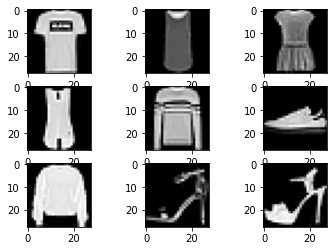We will add an empty color dimension to the dataset. Now the dimensions of the images will be 28 x 28 x 1, so now the images have become three-channel images.

## Python3

 `trainX ``=` `np.expand_dims(trainX, ``-``1``)``testX ``=` `np.expand_dims(testX, ``-``1``)` `print``(trainX.shape)`

## Convolutional Neural Networks (CNN)

Convolutional Neural Network(CNN) is a subclass of an artificial neural network(ANN) which is mostly used for image-related applications. The input for a CNN is an image, and there are different operations performed on that image to extract its important features of it and then decide the weights to give the correct output. These features are learned using filters. Filters help to detect certain image properties such as horizontal lines, vertical lines, edges, corners, etc. As we go deep into the network, the network learns to defect complex features such as objects, face, background, foreground, etc.

CNNs have three main types of layers:

1. Convolutional Layer: This layer is the main layer of CNN. When an image is fed into the convolution layer, a filter or a kernel of varying size but generally of size 3×3 is used to detect the features. The dot product is carried out with the image, and the kernel is the output is stored in a cell of a matrix which is called a feature map or an activation map. Once the operation is done, the filter moves by a distance and then repeats the process. This distance is called a stride. After each convolution operation, a ReLu transformation is applied to the feature map to introduce non-linearity into the model.
2. Pooling Layer: This layer is responsible for reducing the number of parameters in the next layer. It is also known as downsampling or dimensionality reduction.
3. Fully Connected Layer: Neurons in this layer have full connectivity to all the neurons in the preceding layer and the succeeding layer. FC layer helps to map the input with the output.

## Model Training

We will create a straightforward CNN architecture with three convolutional layers followed by three max-pooling layers for this dataset. Convolutional layers will perform the convolutional operation and extract the features, while the max-pooling layer will downsample the features.

## Python3

 `def` `model_arch():``    ``models ``=` `Sequential()``    ` `    ``# We are learning 64``    ``# filters with a kernal size of 5x5``    ``models.add(Conv2D(``64``, (``5``, ``5``),``                      ``padding``=``"same"``,``                      ``activation``=``"relu"``,``                      ``input_shape``=``(``28``, ``28``, ``1``)))``    ` `    ``# Max pooling will reduce the``    ``# size with a kernal size of 2x2``    ``models.add(MaxPooling2D(pool_size``=``(``2``, ``2``)))``    ``models.add(Conv2D(``128``, (``5``, ``5``), padding``=``"same"``,``                      ``activation``=``"relu"``))``    ` `    ``models.add(MaxPooling2D(pool_size``=``(``2``, ``2``)))``    ``models.add(Conv2D(``256``, (``5``, ``5``), padding``=``"same"``,``                      ``activation``=``"relu"``))``    ` `    ``models.add(MaxPooling2D(pool_size``=``(``2``, ``2``)))``    ` `    ``# Once the convolutional and pooling``    ``# operations are done the layer``    ``# is flattened and fully connected layers``    ``# are added``    ``models.add(Flatten())``    ``models.add(Dense(``256``, activation``=``"relu"``))``    ` `    ``# Finally as there are total 10``    ``# classes to be added a FCC layer of``    ``# 10 is created with a softmax activation``    ``# function``    ``models.add(Dense(``10``, activation``=``"softmax"``))``    ``return` `models`

Once the model architecture is defined, we will compile and build the model.

## Python3

 `model ``=` `model_arch()` `model.``compile``(optimizer``=``Adam(learning_rate``=``1e``-``3``),``              ``loss``=``'sparse_categorical_crossentropy'``,``              ``metrics``=``[``'sparse_categorical_accuracy'``])` `model.summary()`

We use Adam optimizers in most CNN architectures because it is very efficient on larger problems and helps us achieve correct weights and learning rates with minimum loss.  The summary of the model is as follows.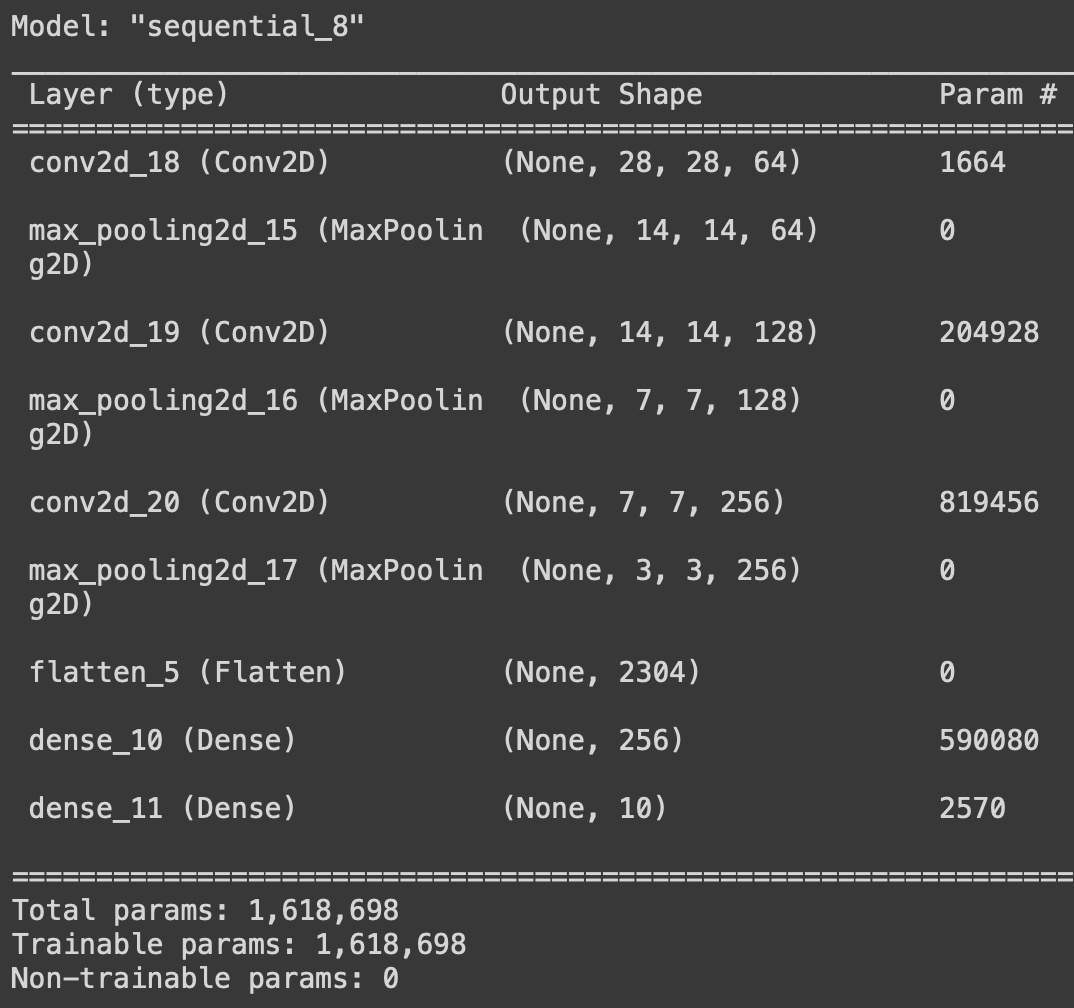Once all the model parameters are set, the model is ready to be trained. We will train the model for ten epochs, with each epoch having 100 steps.

## Python3

 `history ``=` `model.fit(``    ``trainX.astype(np.float32), trainy.astype(np.float32),``    ``epochs``=``10``,``    ``steps_per_epoch``=``100``,``    ``validation_split``=``0.33``)`

Let us save the model.

## Python3

 `model.save_weights(``'./model.h5'``, overwrite``=``True``)`

## Model Analysis

In this section, we will plot some graphs related to accuracy and loss to evaluate model performance. First, we will see the accuracy and plot the loss.

## Python3

 `# Accuracy vs Epoch plot``plt.plot(history.history[``'sparse_categorical_accuracy'``])``plt.plot(history.history[``'val_sparse_categorical_accuracy'``])``plt.title(``'Model Accuracy'``)``plt.ylabel(``'Accuracy'``)``plt.xlabel(``'epoch'``)``plt.legend([``'train'``, ``'val'``], loc``=``'upper left'``)``plt.show()`

Output: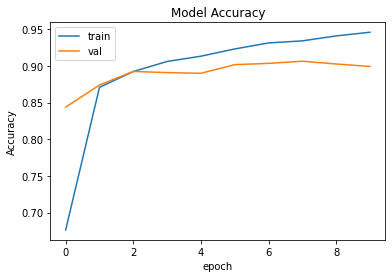## Python3

 `# Loss vs Epoch plot``plt.plot(history.history[``'loss'``])``plt.plot(history.history[``'val_loss'``])``plt.title(``'Model Accuracy'``)``plt.ylabel(``'loss'``)``plt.xlabel(``'epoch'``)``plt.legend([``'train'``, ``'val'``], loc``=``'upper left'``)``plt.show()`

Output: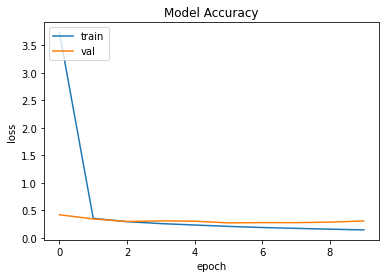To make the predictions call the predict() function on the model and pass the image into it. To perform the prediction, we will first create a list of labels in order of the corresponding output layer of the CNN. The predict() function will return the list of values of probabilities that the current input belongs probably belongs to which class. Then by using the argmax(), we will find the highest value and then output the correct label.

## Python3

 `# There are 10 output labels for the Fashion MNIST dataset``labels ``=` `[``'t_shirt'``, ``'trouser'``, ``'pullover'``, ``'dress'``, ``'coat'``,``          ``'sandal'``, ``'shirt'``, ``'sneaker'``, ``'bag'``, ``'ankle_boots'``]``# Make a prediction``predictions ``=` `model.predict(testX[:``1``])``label ``=` `labels[np.argmax(predictions)]` `print``(label)``plt.imshow(testX[:``1``][``0``])``plt.show()`

Output: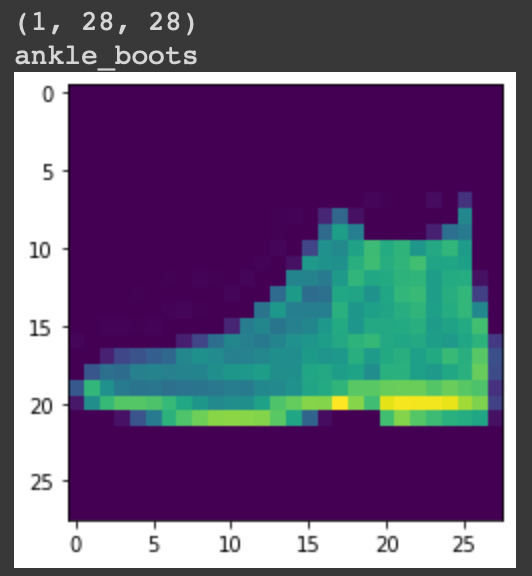Hence we have successfully performed image classification on the fashion MNIST dataset.

My Personal Notes arrow_drop_up